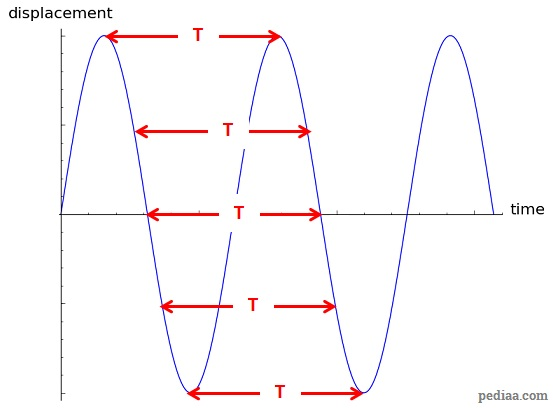# Difference Between Wavelength and Period

## Main Difference –Wavelength vs. Period

Wavelength and period are two different, but related properties of waves. The main difference between wavelength and period is that the wavelength is the shortest distance between two successive points on a wave that are in phase while period is the time taken for a complete oscillation to take place at a given point.

## What is Wavelength

Waves consist of oscillations. If you wiggle one end of a piece of rope up and down as shown in the diagram below, the “wiggles” travel along the rope. In order to transport a wiggle along the rope, each particle on the rope should move up and down. For instance, at the time shown on the diagram below, the particle at A is at the highest position (peak) of its up-down motion. Since the wave is traveling to the right, a particle that is a little to the right of A should be on its way up (so that a little while later, it would be at a peak). This is the case for a particle at B. A particle at C would also be on its way up, a little faster than the particle at B (it would slow down to the speed of B when it reaches that same level).Making waves on a rope

A particle at A’ is also at the peak position. A particle at B’ is on the same stage (or “phase”) of its up-down motion as the particle at B. Similarly, the particles at C and C’ are also at the same stage of their up-down motion. The shortest distance between two adjacent points on a wave that are in the same phase is called a wavelength. A wavelength is often denoted by the greek letter lambda ($\mathbf{\lambda}$). So for this diagram, the distances$\overline{AA'}=\overline{BB'}=\overline{CC'}=\lambda$. This distance is marked on the diagram in green arrows. Note that the distance between two peaks or troughs on a wave is one wavelength.

In terms of a longitudinal wave that is made of compressions and rarefactions, the wavelength is equal to the shortest distance between two adjacent compressions or rarefactions. This is shown in the diagram below:Wavelength in a longitudinal wave

Electromagnetic waves do not consist of particles which are physically oscillating in order to transmit it. Therefore, for electromagnetic waves, the wavelength is the shortest distance between two points whose the electric fields are at the same phase. In general, if you plot a graph of the displacement as a function of position, then the distance between two peaks or two troughs on the graph would represent one wavelength:Wavelength depicted on a graph of displacement vs. position

Since wavelength is a measurement of length, it is measured in metres.

## What is Period

As mentioned before, waves consist of oscillations. The time taken to complete one oscillation is called the period. Period is often denoted as$T$. If you consider the displacement of an individual point over an interval of time and plot a graph of how the displacement varies as a function of time, then one period is represented by the distance between two peaks or two troughs. This is shown in the graph shown below. Since period measures an interval of time, it is measured in units of seconds.Period depicted on a graph of displacement vs. time

The reciprocal of the period is known as the frequency ($\mathbf{f}$). That is,$f=\frac{1}{T}$

Frequency gives the number of complete oscillations per unit time, and it is measured in units of hertz (Hz). For example, the frequency of the musical note “middle C” is about 261.6 Hz. This means that in order to produce this note, particles must vibrate so that each complete vibration of one of these particles takes$\frac{1}{261.6}=0.003823\:\mathrm{s}=3.823\:\mathrm{ms}$.

The period of a wave and its wavelength are related by the speed of the wave. During one period, the wave travels a distance of one wavelength (the position of peaks and troughs at a given time on a wave would be identical to the position of peaks and troughs one period later—each peak has travelled to the position that was occupied by the previous peak one period ago). Therefore, if the speed of the wave is$v$, then:$v=\frac{\lambda}{T}$

## Difference Between Wavelength and Period

### Definition

Wavelength is the shortest distance between two successive points on a wave whose oscillations are in phase.

Period is the time taken to complete one full oscillation.

### Units

Wavelength is a measurement of a distance. Hence, it has SI units of metres.

Period is a measurement of an interval of time. Hence, it has SI units of seconds.

### Depiction on a Graph

Wavelength is given by the distance between two successive peaks/troughs in a displacement vs. position graph.

Period is given by the distance between two successive peaks in a displacement vs. time graph.

Image Courtesy

“Wave in a rope.” by CK-12 Foundation (File:High School Chemistry.pdf, page 178) [CC BY-SA 3.0], via Wikimedia Commons (Modified)

“4th overtone or 5th harmonic. random molecular representation” by Lookang (Own work) [Public Domain], via Wikimedia Commons (Modified)